# How to convert time to decimals in Excel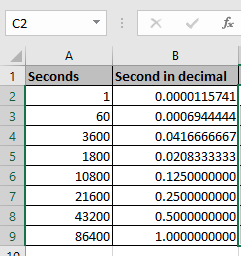In this article, we will learn How to convert time to decimals in Excel.

Scenario:

Excel Stores Time in General number format. So to convert the number in decimals to time format. We need to do this process using mathematical calculation or choose from the format cell option.

Here we need to know that excel To convert seconds in decimal format to a proper Excel time, divide by 86400.

 1 second = 1/86400 = 0.0000115741 = 0:01

Number formats in Excel

There are many number formats in excel. Some of the common uses are shown below.

1. Date
2. Time
3. Number
4. Currency
5. Account
6. Scientific
7. Fraction
8. Decimals.

You can switch from one form to another using the Format cells box.

Example :

All of these might be confusing to understand. Let's understand how to use the function using an example. Here we select the time values and Change format of cells from the Format cell dialog box as shown below. You can open format dialog box using three ways.

• Shortcut Ctrl + 1
• Go to Home > Number > more Formats.
• Right click cell and select Format cells.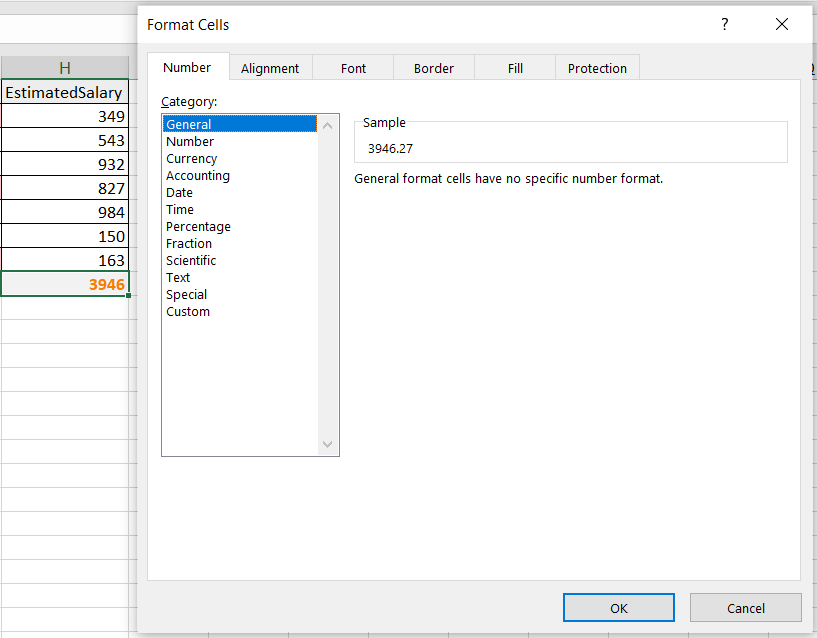Alternate way using Mathematical formula

Here we need to convert time value to decimals using a simple division formula.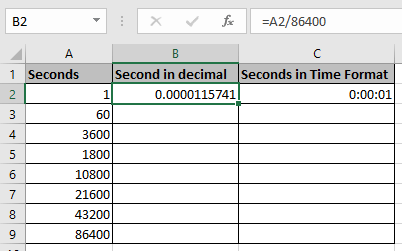First, convert all the seconds to decimals and then convert them to Time format.

Use the formula

 =A3/86400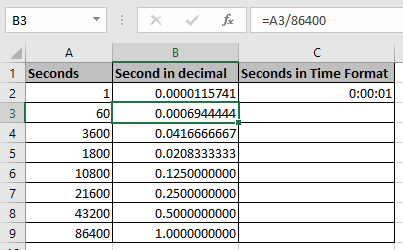As you can see, we have the seconds in decimal format for the time being.

Now I will pick the values and paste them in the Column and change their cell format to Time format.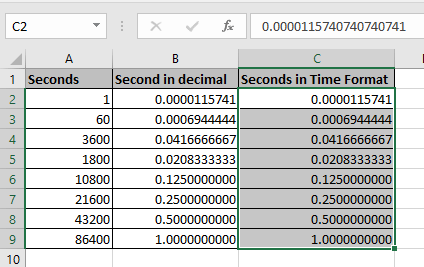Select the cells needed to change the format of the cells.

Then Use Ctrl + 1 to open the format cell dialog box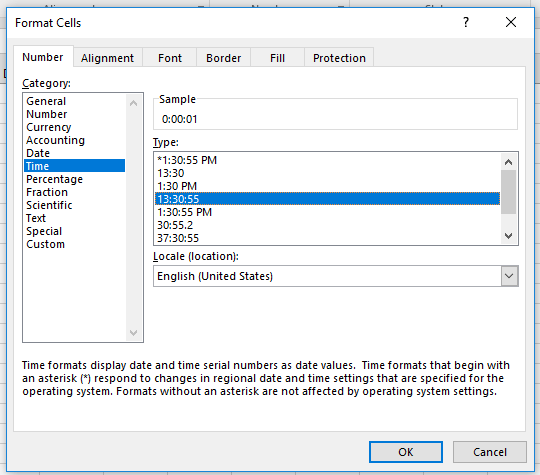Select the Time from category and then select the time format you wish. In this case we are considering 00:00:00(hh:mm:ss).

Click Ok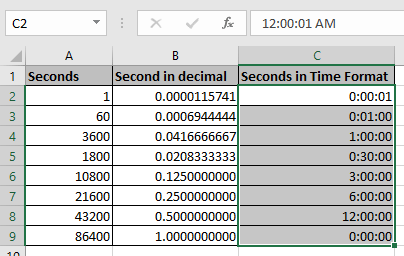As you can see the time format for the seconds(in decimals).

You must be noticing why there is 0:00:00 in C9 cell because 86400 seconds equals one day. So time again starts from 0:00:00. But the date will be increased by one if considered.

Here are all the observational notes using the formula in Excel
Notes :

1. Excel stores Date, time, percent, currency in number format.
2. Use the

Hope this article about How to convert time to decimals in Excel is explanatory. Find more articles on calculating values and related Excel formulas here. If you liked our blogs, share it with your friends on Facebook. And also you can follow us on Twitter and Facebook. We would love to hear from you, do let us know how we can improve, complement or innovate our work and make it better for you. Write to us at info@exceltip.com.

Related Articles :

Converts decimal Seconds into time format : As we know that time in excel is treated as numbers. Hours, Minutes, and Seconds are treated as decimal numbers. So when we have seconds as numbers, how do we convert into time format? This article got it covered.

Calculate Minutes Between Dates & Time in Excel : Take the difference between the two and change the format of output using simple formulation in excel.

Calculate hours between time in Excel : Subtracting two values results into a value. View the result in hours format in Excel using mathematical formula.

How to Convert date to text in Excel : In this article we learned how to convert text into date, but how do you convert an excel date into text. To convert an excel date into text we have a few techniques.

Extract days between dates ignoring years in Excel : count the days between the two dates using different functions and mathematical operation in Excel.

Popular Articles :